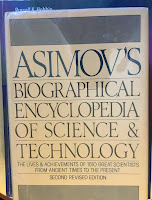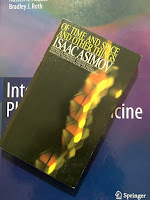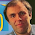## Friday, February 2, 2018

### Gauss and the Method of Least Squares

Intermediate Physics for Medicine and Biology: Gauss and the Method of Least SquaresAsimov’s Biographical Encyclopedia of Science and Technology, by Isaac Asimov.
In Chapter 11 of Intermediate Physics for Medicine and Biology, Russ Hobbie and I discuss fitting data using the method of least squares. This technique was invented by mathematicians Adrien Marie Legendre and Johann Karl Friedrich Gauss. Isaac Asimov describes Gauss’s contributions in Asimov’s Biographical Encyclopedia of Science and Technology.
While still in his teens he [Gauss] made a number of remarkable discoveries, including the method of least squares, advancing the work of Legendre in this area. By this [technique] the best equation for a curve fitting a group of observations can be made. Personal error is minimized. It was work such as this that enabled Gauss, while still in his early twenties, to calculate the orbit for [the asteroid] Ceres.Of Time and Space and Other Things, by Isaac Asimov.
Asimov tells the story of Ceres in more detail in Of Time and Space and Other Things
Giuseppe Piazzi, an Italian astronomer … discovered, on the night of January 1, 1801, a point of light which had shifted its position against the background of stars. He followed it for a period of time and found it was continuing to move steadily. It moved less rapidly than Mars and more rapidly than Jupiter, so it was very likely a planet in an intermediate orbit …

Piazzi didn't have enough observations to calculate an orbit and this was bad. It would take months for the slow-moving planet to get to the other side of the Sun and into observable position, and without a calculated orbit it might easily take years to rediscover it.

Fortunately, a young German mathematician, Karl Friedrich Gauss, was just blazing his way upward into the mathematical firmament. He had worked out something called the “method of least squares,” which made it possible to calculate a reasonably good orbit from no more than three good observations of a planetary position.

Gauss calculated the orbit of Piazzi's new planet, and when it was in observable range once more there was [Heinrich] Olbers [a German astronomer] and his telescope watching the place where Gauss's calculations said it would be. Gauss was right and, on January 1, 1802, Olbers found it.
What is the role for least-squares fitting in medicine and biology? In many cases you want to fit experimental data to a mathematical model, in order to determine some unknown parameters. One example is the linear-quadratic model of radiation damage, presented in Chapter 16 of IPMB. Below is a new homework problem, designed to provide practice using the method of least squares to analyze data on cell survival during radiation exposure.
Section 16.9

Problem 29 ½. The fraction of cell survival, Psurvival, as a function of radiation dose, D (in Gy), is
D Psurvival
0   1.000
2   0.660
4   0.306
6   0.100
8   0.0229
10   0.0037
Fit this data to the linear-quadratic model, Psurvival = eD – βD2 (Eq. 16.29) and determine the best-fit values of α and β. Plot Psurvival versus D on semilog graph paper, indicating the data points and a curve corresponding to the model. Hint: use the least-squares method outlined in Sec. 11.1, and make this into a linear least squares problem by taking the natural logarithm of Psurvival.
Gauss is mentioned often in IPMB. Section 6.3 discusses Gauss’s law relating the electric field to charge, and Appendix I discusses the Gaussian probability distribution (the normal, or bell-shaped curve). Asimov writes
Gauss…was an infant prodigy in mathematics who remained a prodigy all his life. He was capable of great feats of memory and of mental calculation…. Some people consider him to have been one of the three great mathematicians of all time, the others being Achimedes and Newton.

#### 1 comment:

1.In the new homework problem above, the student is told to take the natural log of the survival fraction. Russ Hobbie reminds me that there are dangers to taking the log before doing a linear least squares fit. See section 11.2 in IPMB, where we conclude "The moral: use nonlinear least squares." Unfortunately, making nonlinear least squares homework problems is difficult without using computer software. For a fuller discussion of this point, see Adair, Hobbie, and Hobbie (2010) Single-pool exponential decomposition models: Potential pitfalls in their use in ecological studies. Ecology 91:1225-1236.